# 一、快慢指针的常见算法

## 1、判定链表中是否含有环

boolean hasCycle(ListNode head) {
return false;
}


boolean hasCycle(ListNode head) {
ListNode fast, slow;
while(fast != null && fast.next != null) {
fast = fast.next.next;
slow = slow.next;

if (fast == slow)
return true;
}
return false;
}


## 2、已知链表中含有环，返回这个环的起始位置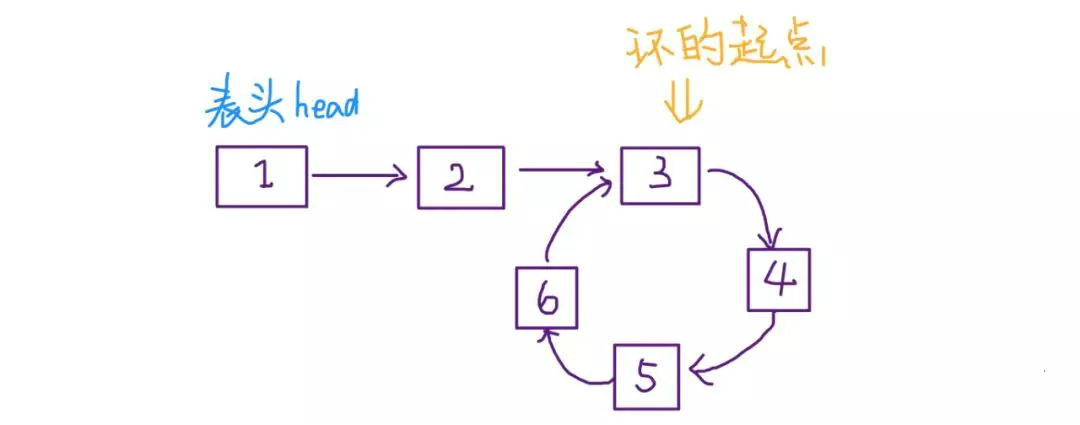ListNode detectCycle(ListNode head) {
ListNode fast, slow;
while (fast != null && fast.next != null) {
fast = fast.next.next;
slow = slow.next;
if (fast == slow)
break;
}

while (slow != fast) {
fast = fast.next;
slow = slow.next;
}
return slow;
}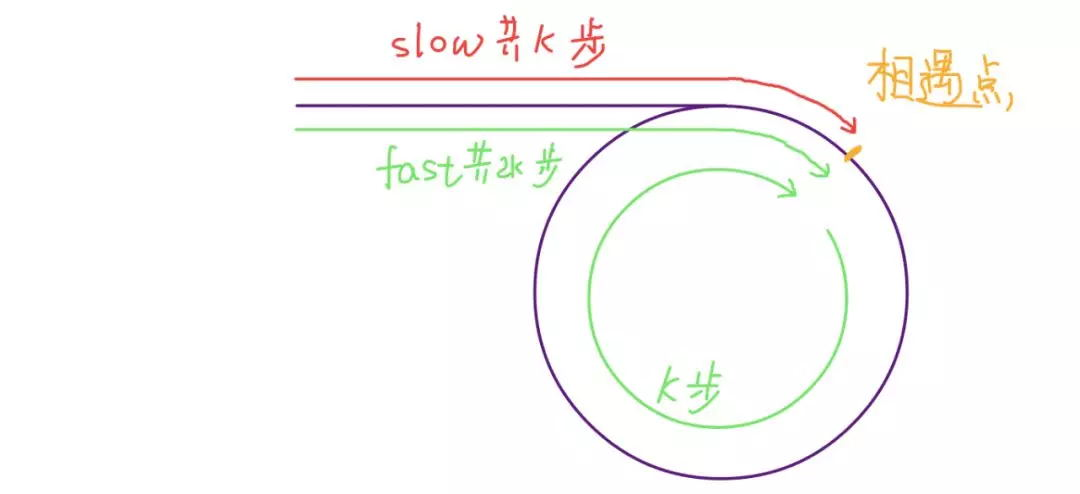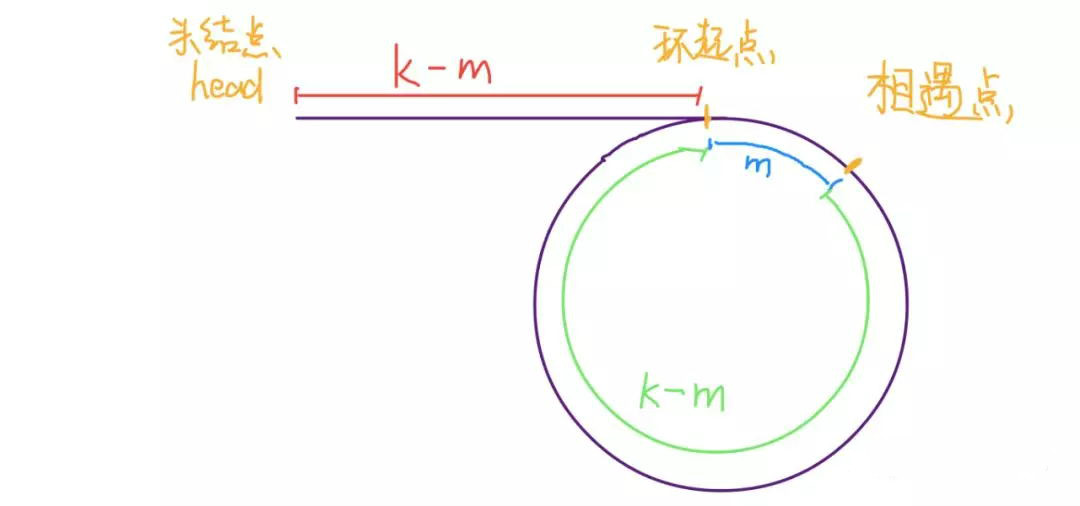## 3、寻找链表的中点

ListNode slow, fast;
while (fast != null && fast.next != null) {
fast = fast.next.next;
slow = slow.next;
}
// slow 就在中间位置
return slow;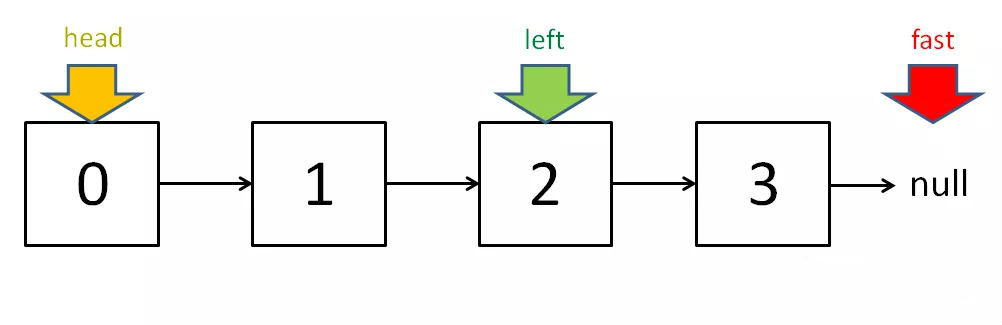## 4、寻找链表的倒数第 k 个元素

ListNode slow, fast;
while (k-- > 0)
fast = fast.next;

while (fast != null) {
slow = slow.next;
fast = fast.next;
}
return slow;


# 二、左右指针的常用算法

## 1、二分查找

int binarySearch(int[] nums, int target) {
int left = 0;
int right = nums.length - 1;
while(left <= right) {
int mid = (right + left) / 2;
if (nums[mid] == target)
return mid;
else if (nums[mid] < target)
left = mid + 1;
else if (nums[mid] > target)
right = mid - 1;
}
}


## 2、两数之和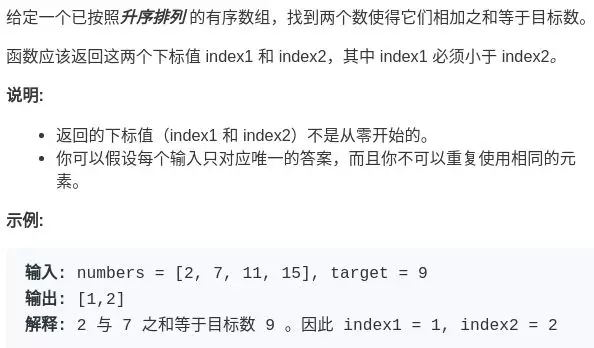## 3、反转数组

void reverse(int[] nums) {
int left = 0;
int right = nums.length - 1;
while (left < right) {
// swap(nums[left], nums[right])
int temp = nums[left];
nums[left] = nums[right];
nums[right] = temp;
left++;
right--;
}
}


## 4、滑动窗口算法

posted @ 2019-06-26 07:53  murphy_gb  阅读(18754)  评论(4编辑  收藏  举报